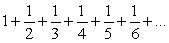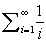# Programming

Question 7
Essay

## Programming Write a static method named sequenceSum that prints terms of the following mathematical sequence: ( also written as )Your method should accept a real number as a parameter representing a limit, and should add and print terms of the sequence until the sum of terms meets or exceeds that limit. For example, if your method is passed 2.0, print terms until the sum of those terms is at ≥ 2.0. You should round your answer to 3 digits past the decimal point. The following is the output from the call sequenceSum(2.0); 1 + 1/2 + 1/3 + 1/4 = 2.083

10+ million students use Quizplus to study and prepare for their homework, quizzes and exams through 20m+ questions in 300k quizzes.

### Computing

Explore our library and get homework help with various study sets and a huge amount of quizzes and questions

440

Study sets

6.6K

Quizzes

455K

Questions

Upload material to get free accessInvite a friend and get free accessSubscribe and get an instant access